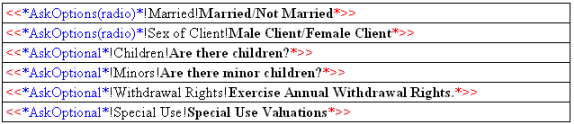Let's assume that, after you have run the Ask Creator, you have this table at the top of your document:Look at the third group "!Children! and the fourth group "!Minors!". Logic dictates that if there are no children, there can be no minor children. It would be better if the answer to that fourth question were automatically set to 'No"  (and, of course, the question not presented) if that situation pertains.

That is done by adding an "If" element to the equation. The structure of an 'If' element is fairly simple. You must be at least a bit familiar with what is called 'If/Then logic', which is simply a standard structure for writing a logic equation of the nature we are using here. Simply put, an 'If/Then' equation would state: "If this situation exists, then do this; else do this."

Once you frame in your head the logic steps, it's easy to move on to the actual equation. In Pathagoras, the "If" statement logic would be "If" the values assigned to "!Children!" is "True", then "Ask" "Are there Minors?"; otherwise, don't ask. Here is the formula in 'code' that Pathagoras can recognize:

Syntax: <<*If* and the final >> are the boundary markers to the equation.

!Children! is the GroupName of the value we are testing.

="True" is the testing value for the GroupName

, concludes the If part of the equation

<<*AskOptional*!Minors!Are there minor children*>> is the 'True' part, and simple tell the program to Ask the question.

, concludes the 'Then' part of the equation

(Since nothing follows the 'Then' part, the Else part is simply 'null.' But in the below example, the value of !Minors is affirmatively set to "False"

Let's say you want to affirmatively set !Minors!         to 'False' when there are no children. Just rewrite the above as follows: